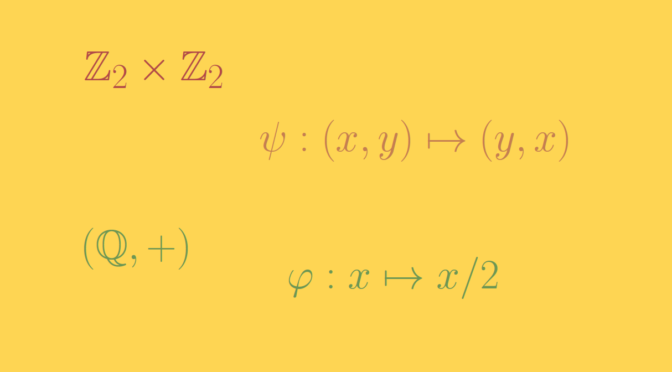# A normal subgroup that is not a characteristic

Let’s $$G$$ be a group. A characteristic subgroup is a subgroup $$H \subseteq G$$ that is mapped to itself by every automorphism of $$G$$.

An inner automorphism is an automorphism $$\varphi \in \mathrm{Aut}(G)$$ defined by a formula $$\varphi : x \mapsto a^{-1}xa$$ where $$a$$ is an element of $$G$$. An automorphism of a group which is not inner is called an outer automorphism. And a subgroup $$H \subseteq G$$ that is mapped to itself by every inner automorphism of $$G$$ is called a normal subgroup.

Obviously a characteristic subgroup is a normal subgroup. The converse is not true as we’ll see below.

### Example of a direct product

Let $$K$$ be a nontrivial group. Then consider the group $$G = K \times K$$. The subgroups $$K_1=\{e\} \times K$$ and $$K_2=K \times \{e\}$$ are both normal in $$G$$ as for $$(e, k) \in K_1$$ and $$(a,b) \in G$$ we have
$(a,b)^{-1} (e,x) (a,b) = (a^{-1},b^{-1}) (e,x) (a,b) = (e,b^{-1}xb) \in K_1$ and $$b^{-1}K_1 b = K_1$$. Similar relations hold for $$K_2$$. As $$K$$ is supposed to be nontrivial, we have $$K_1 \neq K_2$$.

The exchange automorphism $$\psi : (x,y) \mapsto (y,x)$$ exchanges the subgroup $$K_1$$ and $$K_2$$. Thus, neither $$K_1$$ nor $$K_2$$ is invariant under all the automorphisms, so neither is characteristic. Therefore, $$K_1$$ and $$K_2$$ are both normal subgroups of $$G$$ that are not characteristic.

When $$K = \mathbb Z_2$$ is the cyclic group of order two, $$G = \mathbb Z_2 \times \mathbb Z_2$$ is the Klein four-group. In particular, this gives a counterexample where the ambient group is an abelian group.

### Example on the additive group $$\mathbb Q$$

Consider the additive group $$(\mathbb Q,+)$$ of rational numbers. The map $$\varphi : x \mapsto x/2$$ is an automorphism. As $$(\mathbb Q,+)$$ is abelian, all subgroups are normal. However, the subgroup $$\mathbb Z$$ is not sent into itself by $$\varphi$$ as $$\varphi(1) = 1/ 2 \notin \mathbb Z$$. Hence $$\mathbb Z$$ is not a characteristic subgroup.